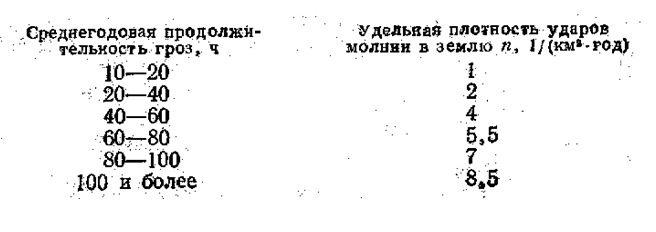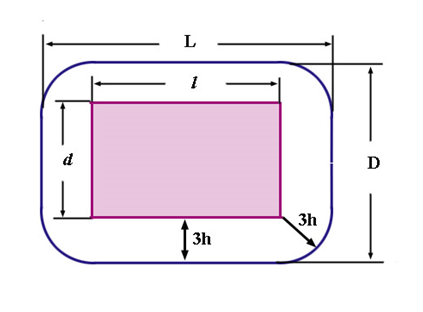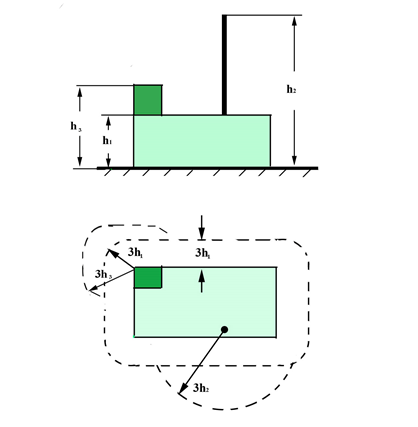# How to count the frequency of lightning strikes into the building?

At a formal approach, it is easy to calculate how often lightning strikes into the house. This will require a contraction area (SCT) of lightnings and their density (nM). We multiply these values ​​and get a precise calculation of the strike rate per year: NM=nMSст

That's the theory, but what we do in practice? In reality, the density is less than one and is represented by a fractional number that is physically meaningless. To get a clear answer, it is necessary to equate the conditional density of the strikes to the unit: Tмол ≈ 1/NM

All the existing manuals fixe the calculated density for 1 square. km per year, that is why, the characteristics of Tмол is calculated in years. For example, nM=0.004, then it is assumed that the frequency of lightning strikes into the building (1: 0.04) on average is once in 25 years.

The word "average" has some special meaning. That does not mean that the lightning will strike 25 years after the construction of the building. This can happen much earlier, even in the same year. Although another outcome is possible: there might be no lightning strike into an object in 90 years. The found time period is average. To make a more realistic calculation of the frequency of lightning strikes, it is necessary to watch a huge number of buildings of the same type for a very long period of time.

## How to find a specific density nM

Reliable lightning protection of residential and public buildings requires calculation of the frequency of strikes. As mentioned above, there is a parameter of specific density in the formula. Here is the chart data (Fig. 1) from the standard AD 34.21.122-87Figure 1. Chart of specific density of lightning strikes into the ground, AD 34.21.122-87

To determine the density, it is necessary to use the map of storms' duration, which is also shown in this authorizing document. We take the average annual duration at the location of the object and look into the chart (Fig. 1). Of course, we will only get the approximate data in the result. The system of registration of the intensity of storm activity with the extension of 200-500 meters will be optimal for the calculations. However, in Russia such a system resource is not wide-spread, whereas in the majority of developed countries, it is successfully used.

## Calculation of the contraction area SCT

The second parameter of the formula for calculating the frequency of lightning strikes into the building is the value of the contraction area. It doesn't require special accuracy of calculations. Based on the statistical data, it was assumed that boundaries of the area will be at the distance of three heights from the external walls of the building. It is not difficult to make a layout (Fig. 2). This is followed by the calculation of the limited area (blue line in Fig. 2), it is possible to use different methods here, e.g., graph paper.Figure 2. Calculation of the contraction area SCT

Often architectural solutions suppose the presence of the building walls of different heights. In these cases, the calculation of the contraction area is held by the size of the highest wall. It is easy to estimate the area in real life when making layouts of the area of ​​all parts of the building, different in height, and then allocating their common border (Fig. 3).Figure 3. Calculation of the contraction area for the presence of the building walls of various heights

Let us note, that such calculations of the sqaure are relevant only for ​​detached houses. Let's say, you are working in lightning protection of buildings, located in the housing system close to each other or located in the suburban village, when there are many diverse objects nearby. Contraction zone at the same time will be superimposed on one another, and that is why the frequency of lightning strikes into each building will be considerably less.

If houses are about the same level of height, it is assumed that the zones are equally distributed between them. If they have a large difference in height, then it will be problematic to calculate all zones superimposed on one another. It should be done with the help of a computer. This is the best calculation method, if the customer requires accurate calculations. Practice shows that there are very few customers who need to know very precisely how often lightning strikes into their home place. Assessment can be considered marginal, mistakes are allowed, due to the inaccurate density of lightning discharges in the Russian Federation.

The material was created on the basis of an article by Professor Eduard Meerovich Bazelyan "What should be protected from a direct lightning strike?"